• ## C++输入字符串的几种方式

万次阅读 多人点赞 2019-05-10 10:20:21
最近有复习到 C++ 基础知识，这里总结下在 C++ 中输入字符串的几种方式，有需要的可以参考： 1、cin >> void Cin1() //输入一个数字 { int a, b; cin >> a >> b; cout << "a + ...

最近有复习到 C++ 基础知识，这里总结下在 C++ 中输入字符串的几种方式，有需要的可以参考：

1、cin >>
<1> 输入一个数字
void Cin1()
{
int a, b;
cin >> a >> b;
cout << "a + b = " << a + b << endl;
}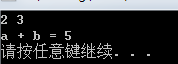<2> 接受一个字符串，遇“空格”、“Tab”、“回车”都结束
void Cin2()
{
char arr;
cin >> arr;
cout << "arr: " << arr << endl;
}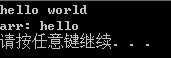2、cin.get()
<1> cin.get(字符变量名)可用来接收一个字符
void Cinget1()
{
char ch;
ch = cin.get();
//cin.get(ch);
cout << "ch = " << ch << endl;
}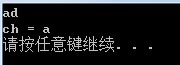<2> cin.get(字符数组名，接收字符数)可用来接收一行字符串，可接收空格,自动接收一个 ‘\0’
void Cinget2()
{
char str = { 0 };
cin.get(str, 20);	//类似于 getline
cout << "str: " << str << endl;
}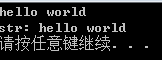<3> cin.get(无参数) 用于舍弃输入流中不需要的字符，或者舍弃回车，弥补cin.get(字符数组名，接收字符数)的不足
void Cinget3()
{
char str = { 0 };
cin.get(str, 20);
cin.get();	//用于吃掉回车，相当于 getchar()
cout << "str: " << str << endl;

cin.get(str, 5);
cout << "str: " << str << endl;
}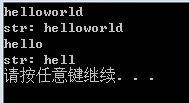3、cin.getline()
<1> 接收一个字符串，可以接收空格并输出,自动接收一个 ‘\0’
void Cingetline1()
{
char arr = { 0 };
cin.getline(arr, 5);
cout << "arr: "  << arr << endl;
}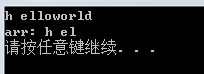<2> cin.getline()实际有三个参数，cin.getline(接收字符串,接收个数，结束字符) 当第三个参数缺省时，系统默认是 ‘\0’
void Cingetline2()
{
char arr = { 0 };
cin.getline(arr, 5, 's');
cout << "arr: " << arr << endl;
}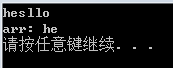<3> 当用在多维数组中时，也可以用 cin.getline(arr[i], 10) 这样的用法
void Cingetline3()
{
char arr = { 0 };
for (size_t i = 0; i < 3; i++)
{
cout << "请输入第"<< i + 1 <<"个字符串: " << endl;
cin.getline(arr[i], 20);
}
cout << endl;
for (size_t i = 0; i < 3; i++)
{
cout << "输出 arr[" << i << "] 的值: " << arr[i] << endl;
}
}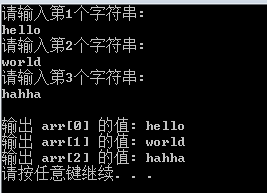4、getline()
<1> 接收一个字符串，可以接收空格并输出，需包含头文件 #include <string> 和 cin.getline() 类似，但是 cin.getline() 属于 iostream 流，而 getline() 属于 string 流，是不一样的两个函数
void Getline()
{
string str;
getline(cin, str);
cout << "str = " << str << endl;
}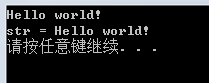5、gets()
<1> gets() 接收一个字符串，可以接收空格并输出，需包含 #include <string>
void Gets()
{
char arr = { 0 };
gets(arr);		//不能写成 arr = gets()
cout << arr << endl;
}

但是上面这段代码在 VS 中无法编译通过，我加了 #include <cstdio> 和 #include <stdio.h> 头文件之后还是无法识别 gets() 函数，后来改成 .c 文件测试的，代码如下：
#include <stdio.h>

void Gets()
{
char arr = { 0 };
gets(arr);		//不能写成 arr = gets()
printf("arr = %s\n", arr);
}

int main()
{
Gets();

system("pause");
return 0;
}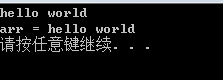6、getchar()
<1> 接收一个字符，需包含 #include <string>
void Getchar()
{
char ch;
ch = getchar();	//不能写成 getchar(ch)
cout << "ch = " << ch << endl;
}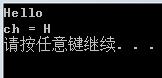展开全文C/C++
• /*编程从键盘输入一个字符串和一个指定字符， 删除该字符串中所有指定的字符，将结果保存到一个新的字符串中并输出*/ #include<stdio.h> #include<string.h> main() { char str,s,c; int i=...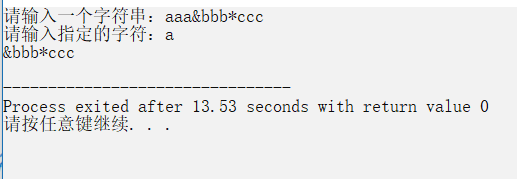/*编程从键盘输入一个字符串和一个指定字符，
删除该字符串中所有指定的字符，将结果保存到一个新的字符串中并输出*/
#include<stdio.h>
#include<string.h>
main()
{	char str,s,c;
int i=0,j=0;
printf("请输入一个字符串：");
gets(str);
printf("请输入指定的字符：");
c=getchar();
while(str[i]!='\0')
{	if(str[i]!=c)/*判断是否为指定的字符*/
{	s[j]=str[i];
j++;
}
i++;
}
s[j]='\0';
puts(s);
}

展开全文• 来源网站： ==== ... 方法一： //输入任意字符串 #include #include #include #define LINE 5 int main() { int i = 0, n = LINE; int l, len; char **str;
来源网站：
==== http://bbs.csdn.net/topics/391045349?page=1#post-399279449

方法一：

//输入任意个字符串
#include <stdio.h>
#include <stdlib.h>
#include <string.h>
#define LINE 5

int main()
{
int i = 0, n = LINE;
int l, len;
char **str;
char buf;    //每个字符串256个字符够吗？

//分配原始内存大小
if (NULL == (str =(char **)malloc(sizeof(char*)*n)))
{
fprintf(stderr, "can not malloc(%d*%d)\n", sizeof(char*), n);
return 1;
}
printf("请输入字符串:(字符串间换行，两个换行结束输入)\n");
while (1)
{
//输入字符串
fgets(buf, 100, stdin);
if ('\n' == buf) break;     //读入空行，结束
//内存已满，扩充
if (i == n)
{
n += LINE;
if (NULL == (str =(char **) realloc(str, sizeof(char*)*n)))
{
fprintf(stderr, "can not realloc(%d*%d)\n", sizeof(char*), n);
return 1;
}
}
len = strlen(buf);
buf[len - 1] = 0;  //去'\n'
if (NULL == (str[i] =(char *)malloc(len - 1)))
{
fprintf(stderr, "can not malloc(%d)\n", len - 1);
return 1;
}
strcpy(str[i], buf);
i++;
}
//打印
printf("\n 你输入的字符串是:\n");
for (l = 0; l < i; l++)
printf("%s\n", str[l]);

system("pause");
return 0;
}
方法二：

#include<stdio.h>
#include<stdlib.h>
#include<malloc.h>
#include<string.h>
int main()
{
char *str=NULL,**strs=NULL,*p;
unsigned int str_size=0,strs_size=0,i;
do
{
char *tmp=(char*)realloc(str,++str_size*sizeof(char));
if(!tmp)
{
free(str);
fputs("out of memory",stderr);
return 1;
}
str=tmp;
str[str_size-1]=getchar();
}while(str[str_size-1]!='\n');
str[str_size-1]=0;
for(p=strtok(str," \t");p;p=strtok(NULL," \t")) {
char **tmp=(char**)realloc(strs,++strs_size*sizeof(char*));
if(!tmp)
{
free(str);
free(strs);
fputs("out of memory",stderr);
return 1;
}
strs=tmp;
strs[strs_size-1]=p;
}
for(i=0;i<strs_size;++i)
printf("%u:%s\n",i+1,strs[i]);
free(strs);
free(str);
return 0;
}


展开全文• python实现统计输入字符中字母，数字，空格和其他字符的个数 # 输入一行字符串，分别统计其中字母，数字，空格和其他字符的个数 word = 0 num = 0 other = 0 space = 0 a = input("请输入一串字符: ") for k in a: ...
python实现统计输入字符中字母，数字，空格和其他字符的个数
# 输入一行字符串，分别统计其中字母，数字，空格和其他字符的个数
word = 0
num = 0
other = 0
space = 0
a = input("请输入一串字符: ")
for k in a:
# 是否为单词
if k.isalpha():
word += 1
# 是否为数字
elif k.isdigit():
num += 1
elif k == ' ':
space += 1
else:
other += 1
print('字母个数是：', word)
print('数字的个数是： ', num)
print('空格的个数是： ', space)
print('其他字符个数是： ', other)

展开全文• JAVA 输入一个字符串，将大写字母改成小写,小写字母改成大写 public class Homework3 { public static void main(String[] args) { Scanner sc = new Scanner(System.in); System.out.println(“请输入字符串：...java
• /*编程实现从键盘输入一串字符串和一个字符，统计该字符串中指定字符出现的个数*/ #include<stdio.h> #include<string.h> main() { char str,c; int i=0,count=0; printf("请输入一个字符串：");...
• **输入任意字符串，输出字符串中数字的个数。 期待结果如下： *****请输入一个字符串：ab34d45 *****字符串中有4个数字。 import java.util.Scanner; public class Demo { public static void main(String[] args) {...java
• 输入一个字符串,把其中的字符按逆序输出。如输入 LIGHT,输出 THGIL。
• [^0]* 或者 [^]* 即可 因为正则中^代表修饰符非, ^0 即非空 简化下来可以直接 [^]javascript
• 逆序输出任意字符串 逆序输出任意字符串 逆序输出任意字符串 逆序输出任意字符串 逆序输出任意字符
• 分析：c语言中字符其实本质和整型一样，输出格式改为%d。 代码如下： #include <stdio.h> int main(){ char c; scanf("%c",&c); printf("%d",c); } 运行结果： ...
• #include &lt;stdio.h&...void reverse(char* s){ // 获取字符串长度 int len = 0; char* p = s; while (*p != 0) { len++; p++; } // 交换 ... int i = 0; char c; while (i &l...
• 正则表达式：几种任意字符的写法 ([^\"]+) ([\s\S]*) ([\d\D]*) ([\w\W]*) 具体不同有待补充正则
• “代表的是除换行以外的任意字符，如果要真正代表任意字符，需要把换行符也加进去，但是经过测试”[.\n]“不生效，可以使用”\s\S"代表，即所有空字符+所有非空字符。 注：为了适用于java程序，上述表达式已把斜杠...
• 在做项目时遇到文本域textarea需要加这样的验证：可以输入任意字符但不能超过200字；解决方法：pattern="^([\w\W]*){1,200}\$" data-bv-message="字数最多200字" required placeholder="最多200字
• System.out.println("请输入一个字符："); ch=(char)buf.read(); if(ch) System.out.println("这是一个控制字符"); else if(ch>='0'&&ch) System.out.println("这是一个数字"); else if(ch>='A'&&ch) System.out....
• @Test public void test13() { Scanner sc = new Scanner(System.in);... //输入字符串 char[] arr = str.toCharArray(); //将字符串转换成字符数组 HashMap&amp;lt;Characte...java
• C语言，从标准输入读入几行输入，并显示在标准输出，每行的前面加上行号。 代码 #include #include int main(void) { int ch; int line_no = 0; int flag = 1; while((ch = getchar()) != EOF) { if ...java
• 问题描述：对任意输入的一段英文，为每个字符编制其相应的赫夫曼编码；并利用该编码为任意输入的0、1序列进行解码． 基本要求：一个完整的系统应具有以下功能： （1）初始化 从终端读入一段英文字符，统计每个字符...
• 输入任意字符串（其中包含数字字符）统计数字字符"0–9"分别出现的次数。 #include<stdio.h> int main() { char s; int i,k, num={0}; //num为计数器 printf("输入串："); gets(s); //也可用 ...c语言
• (.*?) 匹配所有字符串&lt;p class="num"&gt;9033&lt;/p&...会得到num但是如果带换行符会失效，...在字符前加一个\元字符说明举例^匹配整个字符串的起始位置，或者行的起始位置，如果在字符...正则表达式
• 1. 使用%c输出的是字符本身。 2. 使用%d输出的是字符对应的ASCII。 3. 在ASCII中，小写英文字符 = 大写英文字符+32。 4. 还有注意区分单引号''和双引号""的区别。 单引号：字符，是可以进行加减的。 双引号...
• #include&lt;stdio.h&gt;#include&lt;...// 删除字符串中指定字母函数char* deleteCharacters(char * str, char * charSet){ int hash ; if(NULL == charSet) return str; ...删除
• 支持： 1.等长字符串替换 2.长字符串替换为短字符串 3.短字符串替换为长字符串 4.中文替换 .../* 功 能：将str字符串中的oldstr字符串替换为newstr字符串 * 参 数：str：操作目标 oldstr：被替换者...
• 需求：输入一串字符串，统计字符串中每个字符出现的次数** 分析： 1，定义一个需要被统计字符字符串 2，将字符串转化为字符数组，才能拿到每一个字符 3，定义双列集合存储字符串中字符以及字符出现的次数 4，...
• python 字符串的输入和输出 1、字符串的输入 python中提供了input()函数可以用于接收字符串的输入，默认的标准输入是键盘，并且会将输入内容转化为字符串类型，如需将其转化为整型等数据类型，可以使用数据类型转化...python...